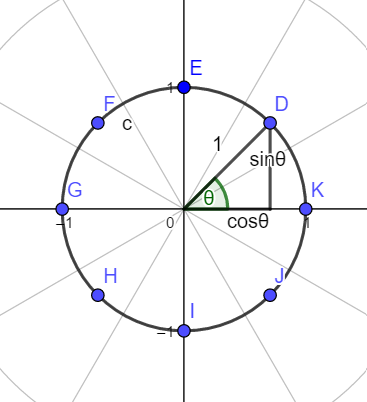# # 多项式

## # 多项式的表示方法

### # 多项式的系数表示法

$f_i$ 表示 $n$ 次多项式$F(x)$ $i$ 次项的系数，则

$F(x)=\sum_{i=0}^nf_ix^i$

# # FFT

## # 复数

### # 复数的运算

• 复数的加法：$(a+bi)+(c+di)=(a+c)+(b+d)i$
• 复数的减法：同理
• 复数的乘法：$(a+bi)(c+di)=ac+adi+bci-bd=(ac-bd)+(ad+bc)i$

## # 单位根

### # 单位根的定义

$n$ 次单位根共有 $n$ 个，它们是：

$\cos\frac{2k\pi}{n}+i\sin\frac{2k\pi}{n}$### # 单位根的性质：

1. $\omega_n^0=1$

1. $\omega_n^n=1$

1. $\omega_{2n}^{2k}=\omega_n^k$

1. $\omega_{n}^{\frac{n}{2}}=-1$

## # 快速傅里叶变换（FFT）

\begin{aligned}F(x)&=f_0x^0+f_1x^1+f_2x^2+\dots\\&=(f_0x^0+f_2x^2+f_4x^4+\dots)+(f_1x^1+f_3x^3+f_5x^5+\dots)\\&=(f_0x^0+f_2x^2+f_4x^4+\dots)+x(f_1x^0+f_3x^2+f_5x^4+\dots)\end{aligned}

，得到

\begin{aligned}F(\omega_n^k)&=L(\omega_n^{2k})+\omega_n^kR(\omega_n^{2k})\\&=L(\omega_\frac{n}{2}^k)+\omega_n^kR(\omega_\frac{n}{2}^k)\end{aligned}

\begin{aligned}F(\omega_n^{k+\frac{n}{2}})&=L(\omega_n^{2k+n})+\omega_n^{k+\frac{n}{2}}R(\omega_n^{2k+n})\\&=L(\omega_\frac{n}{2}^k)-\omega_n^kR(\omega_\frac{n}{2}^k)\end{aligned}

## # 快速傅里叶逆变换

$g_i=F(\omega_n^i)$$G(x)=\sum_{i=0}^ng_ix^i$

\begin{aligned}G(\omega_n^{-k})&=\sum_{i=0}^{n-1}g_i\omega_n^{-ki}\\&=\sum_{i=0}^{n-1}(\sum_{j=0}^{n-1}f_j\omega_n^{ij})\omega_n^{-ki}\\&=\sum_{i=0}^{n-1}\sum_{j=0}^{n-1}f_j\omega_n^{ij}\omega_n^{-ki}\\&=\sum_{j=0}^{n-1}f_j\sum_{i=0}^{n-1}\omega_n^{(j-k)i}\end{aligned}

1. $j=k$ 时，显然这个式子的结果是 $n$
1. $j\neq k$ 时，我们就用到了我们的等比数列求和大法。先看这个等式：
$\sum_{i=0}^{n-1}\omega_n^{(j-k)i}=\sum_{i=0}^{n-1}\omega_n^{(j-k)i}$

$\omega_n^{j-k}\sum_{i=0}^{n-1}\omega_n^{(j-k)i}=\sum_{i=0}^{n-1}\omega_n^{(j-k)(i+1)}$

$(\omega_{n-1}^{j-k}-1)\sum_{i=0}^{n-1}\omega_n^{(j-k)i}=1-\omega_n^{n}$

\begin{aligned}&\ \ \ \ \ \ (\omega_{n-1}^{j-k}-1)\sum_{i=0}^{n-1}\omega_n^{(j-k)i}&=&0\\&\Rightarrow\sum_{i=0}^{n-1}\omega_n^{(j-k)i}&=&0\end{aligned}

\begin{aligned}&\ \ \ \ \ \ G(\omega_n^{-k})&=&\sum_{j=0}^{n-1}f_j\sum_{i=0}^{n-1}\omega_n^{(j-k)i}\\&&=&nf_k\\&\Rightarrow f_k&=&\frac{G(\omega_n^{-k})}{n}\end{aligned}

# # 铭谢

• 感谢@attack 神仙的题解。这篇题解和那篇题解的思路是基本一样的，所以会有很多相似的地方。
2020 02 21 Fri 11:29:36
2020 10 17 Sat 07:00:25